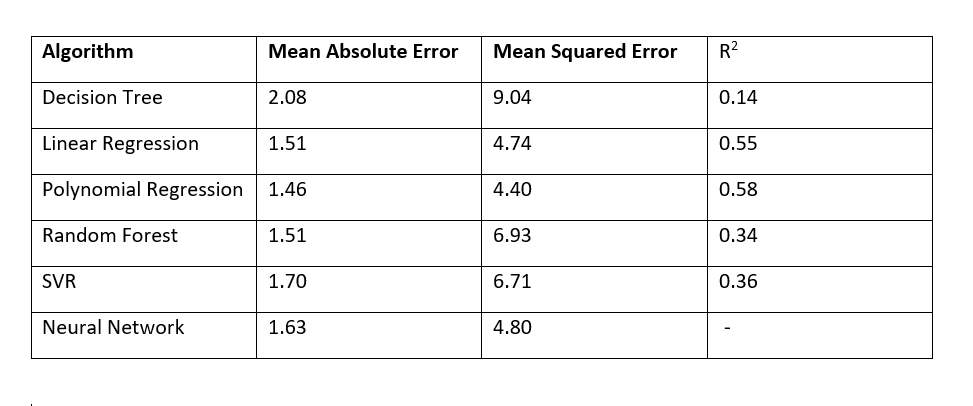# Machine Learning with Abalone

Source: Deep Learning on Medium# Machine Learning with Abalone

At the most basic level, machine learning can be understood as programmed algorithms that receive and analyse input data to predict output values within an acceptable range. As new data is given to these algorithms, they learn to optimize their operations as to improve their performance by developing ‘intelligence’ over time.

In this blog various machine learning algorithms will be compared with the help of Abalone data present in the UCI Repository.

# Predicting Age of Abalone

The age of abalone is determined by cutting the shell through the cone, staining it, and counting the number of rings through a microscope — a boring and time-consuming task. We want to predict the age using different physical measurements which is easier to measure. The age of abalone is ( number of rings +1.5) years.

# Dataset

Sex / nominal / — / M, F, and I (infant)
Length / continuous / mm / Longest shell measurement
Diameter / continuous / mm / perpendicular to length
Height / continuous / mm / with meat in shell
Whole weight / continuous / grams / whole abalone
Shucked weight / continuous / grams / weight of meat
Viscera weight / continuous / grams / gut weight (after bleeding)
Shell weight / continuous / grams / after being dried
Rings / integer / — / +1.5 gives the age in years

In the data set all the columns except ‘Sex’ is numerical type.

`import pandas as pdimport numpy as nppath="/content/drive/My Drive/Machine learning 303L/abalone.csv"df=pd.read_csv(path)print(df.shape)print(df.head())`

# Preprocessing

For the categorical data ‘Sex’ we will do One-Hot-Encoding for ‘M’(Male),‘F’(Female) and ‘I’(Infant).

`df["M"] = np.nandf["F"] = np.nandf["I"] = np.nancolumnName='Sex'for i in range (len(df[columnName])): if df[columnName][i]=='M': df['M'][i]=1 df['F'][i]=0 df['I'][i]=0 elif df[columnName][i]=='F': df['M'][i]=0 df['F'][i]=1 df['I'][i]=0 elif df[columnName][i]=='I' : df['M'][i]=0 df['F'][i]=0 df['I'][i]=1df=df.drop(['Sex'],axis=1)`

For the numerical data we will normalize the data using python libraries. We normalize as we want to bring the data in a particular range.

Rings column will be taken as output column and others as input.

`from sklearn import preprocessingx=df.drop(['Rings'],axis=1)y=df['Rings']x = preprocessing.normalize(x)`

Now we will divide the dataset into training and testing set.

`from sklearn.model_selection import train_test_splitx_train, x_test, y_train, y_test = train_test_split(x, y, test_size = 0.3, random_state = 0)`

# Training different models

Now we will train various models for this regression problem using python libraries. We will compare with the help of Regression metrics namely MAE (mean absolute error),MSE (mean squared error) and (R squared) for comparison of different models.

## Importing libraries

`from sklearn.metrics import mean_squared_errorfrom sklearn.metrics import mean_absolute_errorfrom sklearn.tree import DecisionTreeRegressor from sklearn.metrics import r2_scorefrom sklearn.linear_model import LinearRegressionfrom sklearn.preprocessing import PolynomialFeaturesfrom sklearn.ensemble import RandomForestClassifierfrom sklearn.svm import SVRimport warningswarnings.filterwarnings('ignore')from keras.models import Sequential,model_from_jsonfrom keras.layers import Densefrom keras.optimizers import RMSprop`

## Decision Tree

Decision tree builds regression or classification models in the form of a tree structure. It breaks down a dataset into smaller subsets and the tree is developed subsequently. The final result is a tree with decision nodes and leaf nodes.

`regressor = DecisionTreeRegressor(random_state = 0) regressor.fit(x_train, y_train) y_predicted1 = regressor.predict(x_test)`

## Linear Regression

Linear regression uses a linear model to predict the relationship between two or more variables or factors.

`linearRegressor = LinearRegression()linearRegressor.fit(x_train, y_train)y_predicted2 = linearRegressor.predict(x_test)`

## Polynomial Regression

Polynomial Regression is a form of linear regression in which the relationship between the independent variable x and dependent variable y is modeled as an nth degree polynomial.

`polynomial_features= PolynomialFeatures(degree=2)x_poly = polynomial_features.fit_transform(x_train)x_poly_test = polynomial_features.fit_transform(x_test)model = LinearRegression()model.fit(x_poly, y_train)y_predicted3 = model.predict(x_poly_test)`

## Random Forest

Random Forest is an ensemble method for classification or regression which combines multiple decision trees.

`rf = RandomForestClassifier()rf.fit(x_train,y_train);y_predicted4 = rf.predict(x_test)`

## Support Vector Machine — Regression (SVR)

The Support Vector Regression (SVR) uses the same principles as the SVM for classification: to minimize error, individualizing the hyperplane which maximizes the margin, keeping in mind that part of the error is tolerated

`regressor = SVR(kernel='rbf')regressor.fit(x_train,y_train)y_predicted7 = regressor.predict(x_test)`

## Neural Network

A neural network is a series of algorithms that endeavors to recognize underlying relationships in a set of data through a process that mimics the way the human brain operates.

We will use densely connected 4 layers. The input will contain 10 nodes and output will contain only 1 node. The calculated loss will be MAE.Other activation functions can be used with different number of nodes.

`model = Sequential()model.add(Dense(256, activation='relu', input_shape=(10,)))model.add(Dense(256, activation='relu'))model.add(Dense(256, activation='relu'))model.add(Dense(256, activation='relu'))model.add(Dense(1, activation='relu'))model.summary()model.compile(loss='mean_absolute_error', optimizer='adam', metrics=['mean_absolute_error'])history = model.fit(x_train,y_train,batch_size=100,epochs=5,verbose=1)test=model.evaluate(x_test, y_test, verbose=1)`

# Using Regression Metrics

We are using python libraries for implementing regression metrics.

`mse1=mean_squared_error(y_test, y_predicted1)r21=r2_score(y_test, y_predicted1)mae1=mean_absolute_error(y_test,y_predicted1)`